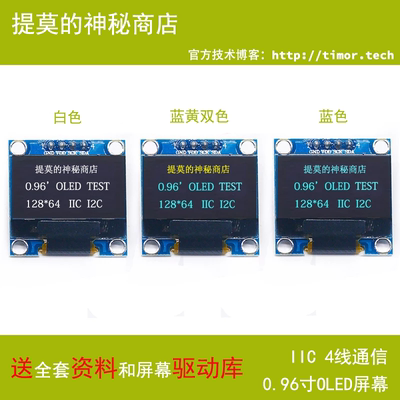# 树莓派使用 Python 驱动 SSD1306 中文字体、图片动画高级教程（IIC/SPI 通信）## 显示中文和指定中文字体

``````# -*- coding: UTF-8 -*-

from luma.core.interface.serial import i2c, spi
from luma.core.render import canvas
from luma.oled.device import ssd1306
from PIL import Image, ImageDraw, ImageFont
import time

serial = spi(device=0, port=0)
device = ssd1306(serial)

font = ImageFont.truetype("GenJyuuGothic-P-Regular.ttf", 16)

with canvas(device) as draw:
draw.rectangle(device.bounding_box, outline="white", fill="black")
draw.text((8, 20), "提莫的神秘商店".decode("UTF-8"), fill="white", font=font)

while (True):
pass

``````

## 显示图片

``````# https://github.com/rm-hull/luma.core/blob/master/luma/core/render.py#L22

def __init__(self, device, background=None, dither=False):
self.draw = None
if background is None:
self.image = Image.new("RGB" if dither else device.mode, device.size)
else:
assert(background.size == device.size)
self.image = background.copy()
self.device = device
self.dither = dither
``````

``````# https://github.com/rm-hull/luma.oled/blob/master/luma/oled/device/__init__.py#L191

def display(self, image):
"""
Takes a 1-bit :py:mod:`PIL.Image` and dumps it to the OLED
display.
:param image: Image to display.
:type image: :py:mod:`PIL.Image`
"""
assert(image.mode == self.mode)
assert(image.size == self.size)

image = self.preprocess(image)

self.command(

buf = bytearray(self._w * self._pages)
off = self._offsets

idx = 0
for pix in image.getdata():
if pix > 0:
idx += 1

self.data(list(buf))
``````

``````# -*- coding: UTF-8 -*-

from luma.core.interface.serial import i2c, spi
from luma.core.render import canvas
from luma.oled.device import ssd1306
from PIL import Image, ImageDraw

serial = spi(device=0, port=0)

device = ssd1306(serial)

im = Image.open("./demo.png").convert(device.mode)

device.display(im)

while (True):
pass

``````

``````assert(image.mode == self.mode)
assert(image.size == self.size)
``````## 制作动画

``````# -*- coding: UTF-8 -*-

from luma.core.interface.serial import i2c, spi
from luma.oled.device import ssd1306
from PIL import Image, ImageDraw, ImageFont
import time

serial = spi(device=0, port=0)
device = ssd1306(serial)

font = ImageFont.truetype("GenJyuuGothic-P-Regular.ttf", 16)

canvas = Image.new(device.mode, device.size)
draw = ImageDraw.Draw(canvas)

while (True):
draw.rectangle(device.bounding_box, outline="white", fill="black")
datestr = time.strftime("%Y年%m月%d日", time.localtime())
timestr = time.strftime("%H:%M:%S", time.localtime())
draw.text((5, 10), datestr.decode("UTF-8"), "white", font)
draw.text((30, 30), timestr, "white", font)
device.display(canvas)

``````